Two Port Network - 1

# Two Port Network - 1 - Electrical Engineering SSC JE (Technical) - Electrical Engineering (EE)

Two Port network

• A pair of terminal through which a current may enter or leave a network is known as a port. Two-terminal devices or element (such as resistors, capacitors, and inductors) result in one-port  networks. 
• Here, we are mainly concerned with the twoport networks. A two-port network is an electrical network with two separate ports for input and output. Thus, a two-port network has two terminal pairs acting as access points. The current entering in the pair leaves the other terminal in the pair. Three-terminal devices such as transistors can be configured into two-port networks. 
• Armed only with the knowledge that the circuit is linear, and the ability to measure the voltage and currents, we will shortly see that it is possible to characterize such a network with a set of parameters that allows us to predict how the network will interact with other networks.

Network parameters 

• Z– parameters 
• Y= parameters 
• ABCD parameters (Transmission parameter) 
• A' B' C' D' parameters (Inverse transmission parameter) 
• h-parameters 
• g-parameters

Z – PARAMETERS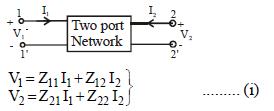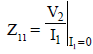....the input driving- point impedance with the output port open-circuited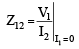...The reverse transfer impedance with the input port open-circuited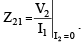.....the forward transfer impedance with the output port open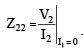.....the output driving point impedance with input port opencircuited

•  The equivalent circuit representation of equation
(i) is in figure below, where Z12 I2 and Z21 I1 are current controlled voltage sources (CCVS) 
• Rewriting equation (i),

V1 = (Z11 –Z12)I1+ Z12 (I1 + I2)
V2 = (Z21 – Z12) I1 + (Z22 –Z12)I+ Z12 (I1 + I2) ...........(ii)

• The equivalent circuit for equation (i) is given as,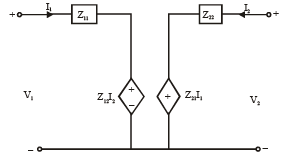• The equivalent circuit for equation (ii) is given as,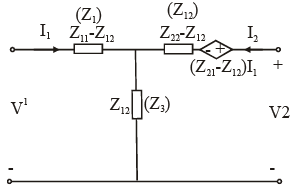Condition of Reciprocity and Symmetry

Reciprocal Network 

• A network must be reciprocal when ratio of response at port 2 to the excitation at port '1' is same as ratio of response at port 1 to the excitation at port 2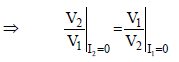Z11 = Z22

Y- PARAMETERS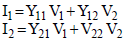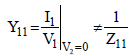.............(Because in Y11 we have short circuit condition whereas in Z11 we have open cuicuit condition) input driving point admittance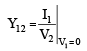.....(reverse transfer admittance)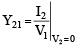....(forward transfer admittance)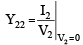...(o utput driv in g po in t admittance)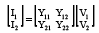Equivalent Circuit Y-parameter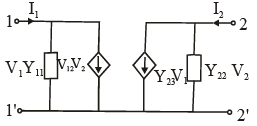• Rewriting equation of Y-parameters

I1 = (y11 + Y12) V1 – Y12 (V1-V2)

I2 = (Y23 – Y12) V1 + (Y22 + Y12)V2 – Y12 (V2–V1)

For this equivalent II–network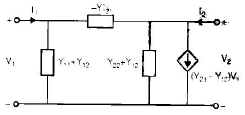For reciprocal network Y12 = Y21
For sysmmetrical network Y11  = Y22

TRANSMISSION PARAMETERS (ABCD)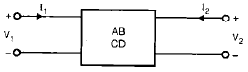V1 = AV2 + B (–12)
I1 = CV2 + D (–I2)

The transmission parameters are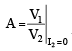..(i.e. the reverse voltage ratio with the receiving end open circuited)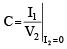..(i.e. the transfer admittance with the receiving end open-circuited)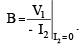....(i.e. the transfer impedance with the receiving end short-circuited )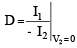...(i.e. the  reverse  current ratio with the receiving end short circuited.)

Condition for Reciprocity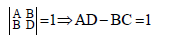Condition for Symmetry
A=D

INVERSE TRANSMISSION PARAMETERS
V2 = A'V+ B' (–I1)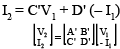The inverse transmission parameter can be defined as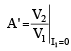...(forward voltage ratio with sending end open circuited)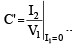...(transfer admittance with sending end open circuited)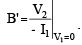....(transfer impedance with sending end short circuited)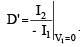...(forward current ratio with sending end short circuited)

Condition of Reciprocity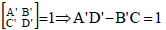Condition for Symmetry

A' = D'

The document Two Port Network - 1 | Electrical Engineering SSC JE (Technical) - Electrical Engineering (EE) is a part of the Electrical Engineering (EE) Course Electrical Engineering SSC JE (Technical).
All you need of Electrical Engineering (EE) at this link: Electrical Engineering (EE)

## FAQs on Two Port Network - 1 - Electrical Engineering SSC JE (Technical) - Electrical Engineering (EE)

 1. What is a two-port network in electrical engineering?Ans. A two-port network is a linear electrical network that has two pairs of terminals. It is commonly used to describe the behavior of electrical systems, such as amplifiers and filters. Each port consists of two terminals, and the network can be characterized by its input and output impedance or admittance.
 2. How can a two-port network be represented mathematically?Ans. A two-port network can be represented mathematically using a set of four equations known as the scattering parameters (S-parameters). These equations describe the relationship between the incident and reflected waves at each port. The S-parameters are typically represented as a matrix and provide information about the network's gain, impedance matching, and transmission characteristics.
 3. What are the applications of two-port networks?Ans. Two-port networks have a wide range of applications in electrical engineering. They are commonly used in the design and analysis of amplifiers, filters, transmission lines, and communication systems. Two-port networks also find applications in signal processing, radar systems, and antenna design. Their ability to describe the behavior of complex electrical systems makes them a valuable tool in engineering and circuit design.
 4. How can we analyze a two-port network?Ans. There are different methods to analyze a two-port network, depending on the information required. One common approach is to use the S-parameters to calculate the network's gain, impedance matching, and transmission characteristics. Another method is to use circuit analysis techniques, such as mesh or nodal analysis, to solve for the voltages and currents within the network. Additionally, computer-aided design (CAD) tools and simulation software can be used to analyze and optimize the performance of two-port networks.
 5. What is the significance of input and output impedance in a two-port network?Ans. The input and output impedance of a two-port network play a crucial role in determining its performance and compatibility with other electrical systems. The input impedance determines how well the network can accept signals from the source, while the output impedance determines how well it can deliver signals to the load. Matching the impedance between the source, network, and load is important for maximum power transfer and minimizing signal distortion. By analyzing the input and output impedance, engineers can design and optimize two-port networks for specific applications.

## Electrical Engineering SSC JE (Technical)

2 videos|75 docs|40 tests

## Electrical Engineering SSC JE (Technical)

2 videos|75 docs|40 tests
Signup to see your scores go up within 7 days! Learn & Practice with 1000+ FREE Notes, Videos & Tests.
10M+ students study on EduRev
Track your progress, build streaks, highlight & save important lessons and more!(Scan QR code)
Related Searches

,

,

,

,

,

,

,

,

,

,

,

,

,

,

,

,

,

,

,

,

,

;